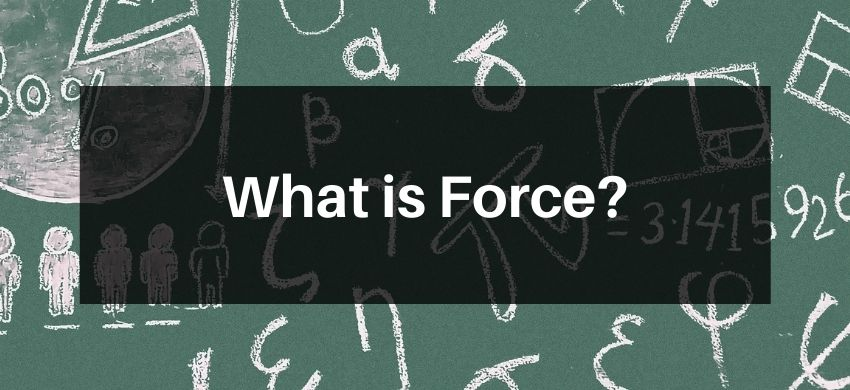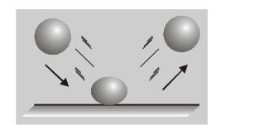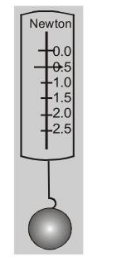Most Affordable JEE | NEET | 8,9,10 Preparation by Kota's Top IITian Doctor Faculties

# What is Force?## Force

A force is a push or a pull.

 Since push or a pull has both magnitude and direction, so that force is a vector quantity.

 Forces occur in pairs. If object $A$ exerts a force on object $B$, then $B$ also exerts a force on $A$. For example, when a bat strikes a ball the bat exerts a force on the ball, but the ball exerts a force on the bat also.

 A force can cause an object to accelerate. If you kick a foot ball the ball's velocity changes while your foot is in contact with it.A force can deform an object. As you can see from fig. The ball when hits the floor, is deformed by the contact force exerted on it by the floor. The floor is deformed too, but since it is harder than the ball, its deformation is not as noticeable.

Property 4, that a force causes an object to be deformed is often used to measure a force. This is the principle of a spring scale.A spring scale indicates the amount of the spring is stretched or compressed. The magnitude of this force is proportional to the amount of the spring is stretched (or compressed), and the direction of the force is along the spring. The scale may be calibrated to read in newton (N), units of force in SI system is newton and in C.G.S. system is dyne.

1 newton $=10^{5}$ dyne. $1 \mathrm{~kg} w \mathrm{t}=\mathrm{g}$ newton.

Dimensional formula of force is $\mathrm{M} \mathrm{L} \mathrm{T}^{-2}$